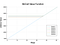# New QuantEcon Julia Packages

About a week ago, we were excited to announce the result of an overhauled set of Julia lectures.

As a part of that process, we created some new Julia packages to be described here. We hope that you’ll find these useful in your own quantitative Julia work.

# Expectations.jl

A common problem in economics is, given a random variable X, take its expectation (or the expectation of some function f(X)). For example, the outcomes of the McCall model depend on the expectation of the wage distribution.These McCall value functions were computed using Expectations.jl. The value functions start increasing when workers decide the payoff of taking a job exceeds the expected payoff of waiting for a better job.

Julia has excellent support for creating and sampling from distributions:

`using Distributions, Statistics, LinearAlgebradist = Exponential()rand(dist, 4)    # gives me 4 values from dist`

If you wanted to take an expectation (say, of where x follows the exponential distribution with θ = 1.0), you might have to do something like this (i.e., a Monte Carlo approach):

`samples = rand(dist, 10^6)mean(samples.^3)`

The Expectations.jl package makes this process a lot easier (and faster!), by defining callable objects (formally “expectations operators,” as they act on functions) which wrap around the distributions objects.

For example, instead of the above, all we need is:

`E = expectation(dist)E(x -> x^3)`

Note that the expectations object here is persistent; once defined, we can reuse it:

`E(x -> x)             # expectation of xE(x -> x^2 * sin(x))  # some complicated function`

Digging into the object, we see something like:

`nodes(E)     # a 32-element array (size is customizable)weights(E)   # a 32-element array (as above)E(x -> x^3)  # mathematically: dot(weights(E), nodes(E).^3)`

A curious reader might wonder where these nodes and weights come from. We compute them for common distributions (`Normal`, `LogNormal`, `Beta`, `Gamma`, `Exponential`) using optimal distribution-specific algorithms (see https://en.wikipedia.org/wiki/Gaussian_quadrature).

Outside of those distributions, we offer a few fall backs:

• Generic Gauss-Legendre quadrature for compact continuous distributions.
• The`qnwdist`algorithm by Spencer Lyon, which chooses its nodes by taking a linear grid along quantiles (that is, by picking some uniform grid on `[0, 1]`, and then picking the nodes to satisfy `F(node)= gridpoint`).
• Trapezoidal (Newton-Cotes) integration for compact distributions (continuous or discrete).
• Exact expectations for finite discrete distributions.

You can also use these objects as linear operators (that is, multiplied with vectors):

`E = expectation(dist)x = nodes(E)E * f.(x) # f is some function we define`

This allows economists to further exploit one of Julia’s key features — code which looks like whiteboard math. For example, the plot above was generated with:

`T(v) = max.(w / (1 - β), c + β * E*v)`

where the `E*v` is the expectation operator for a wage distribution acting on a vector of values `v(w)` (if that sounds puzzling, read the lecture!)

Lastly, let’s grab some quick benchmarking information*:

`@btime mean(rand(dist, 10^6))6.145 ms (4 allocations: 7.63 MiB)@btime E = expectation(dist) # create the object 68.275 μs (44 allocations: 21.06 KiB)@btime E(x -> x^3) # use the object 329.081 ns (5 allocations: 464 bytes)`

This shows us that:

• Once we’ve defined an expectations object, the`Expectations.jl` implementation wins by several orders of magnitude (recall that nanoseconds are 1e-9, microseconds are 1e-6 , and milliseconds are 1e-3).
• The cost of creating the expectations object itself is not very high, but may not be worth it for distributions you don’t intend to reuse.

*Note that in Julia, benchmarking with globals (i.e., without interpolation markers `\$`) should generally be avoided. We’ve left them out for clarity’s sake, since the general story doesn’t change.

# InstantiateFromURL.jl

This package solves a persistent problem with Jupyter notebooks (which we love at QuantEcon), which is that notebooks aren’t executable on users’ machines unless they happen to have the right dependencies (and versions!) installed.

What we’ve done is allow Julia dependency information (for any Julia project) to live in a GitHub repo, to be downloaded and used by the notebook as needed.

For example, in the QuantEcon Julia lectures we have

`using InstantiateFromURLactivate_github(“QuantEcon/QuantEconLecturePackages”, tag = “v0.9.5”)`

The call above will:

1. Create a `.projects` directory in the notebook’s location, if it doesn’t already exist.
2. Check if that directory already has version `0.9.5` of this repo. If it does, just make it the active environment (i.e., use package versions specified by the files in the repo). This similar to a `virtualenv` in Python.
3. If it doesn’t, then: (a) download the dependency files (which in 1.0 are encoded in TOML — a lightweight, human-readable format), (b) “instantiate” them, or make sure that the packages they refer to are actually installed on the machine, and then (c) activate as above.

There are no requirements on the repo other than that it contain a `Project.toml` and (preferably) a `Manifest.toml`, which will pin down exact versions (the `Project.toml` is just a list of dependency names).

# Acknowledgements

QuantEcon is supported by NumFOCUS and the Alfred P. Sloan Foundation. I worked on these packages as part of Jesse Perla’s team at the UBC Vancouver School of Economics.

Written by

Written by

## Arnav Sood

#### Econ Pre-Doc @ UBC